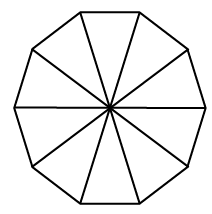### Home > INT2 > Chapter 8 > Lesson 8.2.1 > Problem8-27

8-27.Joey uses ten congruent isosceles triangles to create a regular decagon.

1. What are the three angle measures of one of the triangles? Explain how you know.

See the steps for problem 8-26.

2. If the area of each triangle is $14.5$ square inches, then what is the area of the regular decagon?

The total area is equal to the area of all ten of these triangles.